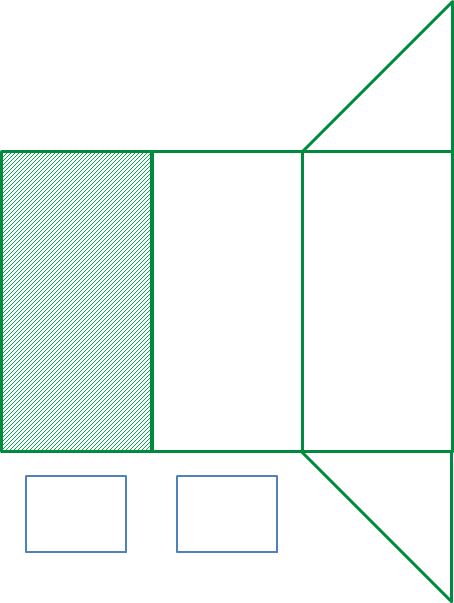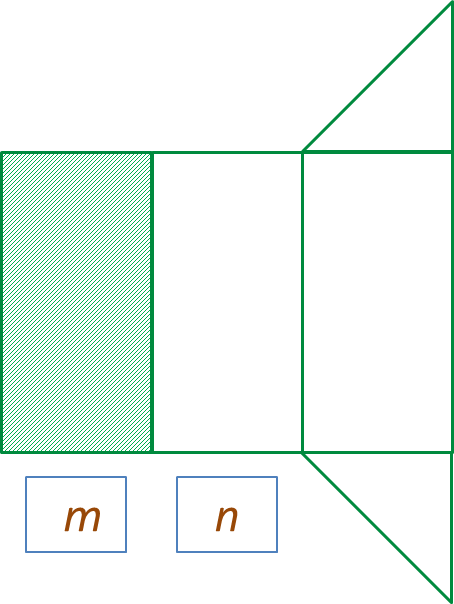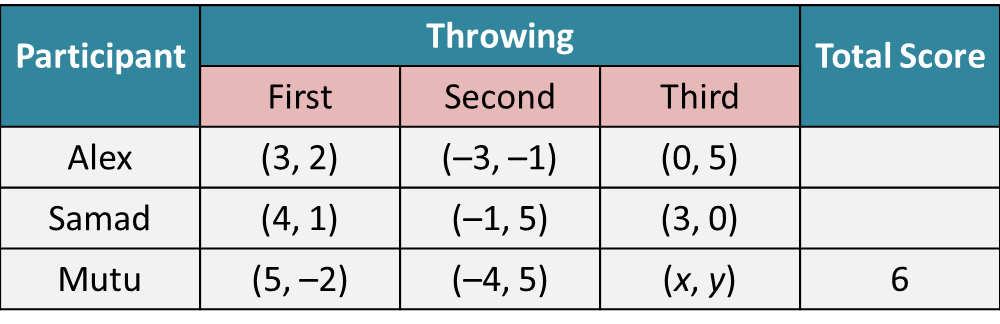# PT3 Mathematics 2016, Question 7

 
Question 7 (a):
Diagram 7.1 shows a right prism.(i) In the answer space, Diagram 7.2 is the net of the right prism.
Fill in the box with m and n on the net correspond to the Diagram 7.1.(ii) Mark (\/) for the correct net and (x) for the incorrect net of the right prism.Solution:
(i)(ii)Question 7 (b):
  
Diagram 7.3 shows a target board which consists of three regions with different scores. Alex, Samad and Mutu are the participants and they are required to make three throws from the fixed distance of 3 metres to the target board.(i) Table 7 shows an incomplete results of the game.
  Complete Table 7.(ii) Based on Table 7, find the coordinates (x, y) if its distance from the origin is 3 units.
  
  Solution:
  
(i)
  Score for Alex = 2 + 1 + 3 = 6
  Score for Samad = 2 + 3 + 2 = 7
  
  (ii)
  Point (x, y) is in the region of 1 point.
  Point (x, y) is 3 units from the origin.
  (x, y) = (–3, 0)
  
  
  
  
   
  
     
Question 7 (c):
  
Tammy lives on the 13th floor in an apartment. She is going 15 floors down to the basement parking to take the present that she left in her car. Then, she goes up to Sandy’s house to celebrate a birthday party.
  Meanwhile, Issac lives on the 10th floor. Then, he goes to another 6 floors up to invite his cousin and goes down to Sandy’s house.
  If the number of floors for Tammy’s movement and the number of floors for Issac’s movement are the same, find the floor for Sandy’s house.
  
  Solution:
  
Tammy’s movement = Issac’s movement
  13 – 15 + x = 10 + 6 – x
  
–2 + x = 16 – x
  
x + x = 18
  x = 9
  
  Sandy’s house
  = 13 – 15 + 9
  = 7
  
  Sandy’s house at level 7.